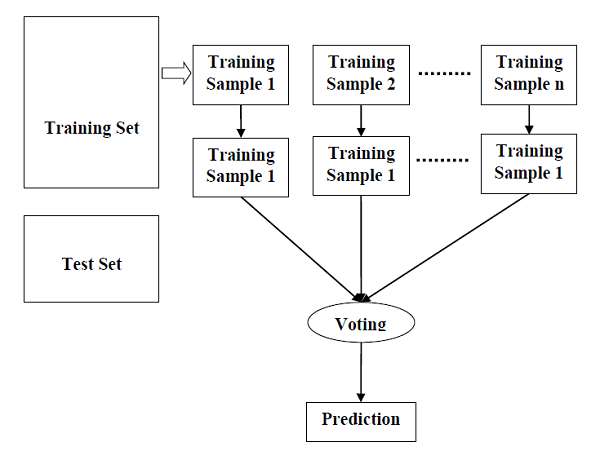# Classification Algorithms - Random Forest

## Introduction

Random forest is a supervised learning algorithm which is used for both classification as well as regression. But however, it is mainly used for classification problems. As we know that a forest is made up of trees and more trees means more robust forest. Similarly, random forest algorithm creates decision trees on data samples and then gets the prediction from each of them and finally selects the best solution by means of voting. It is an ensemble method which is better than a single decision tree because it reduces the over-fitting by averaging the result.

## Working of Random Forest Algorithm

We can understand the working of Random Forest algorithm with the help of following steps −

• Step 1 − First, start with the selection of random samples from a given dataset.

• Step 2 − Next, this algorithm will construct a decision tree for every sample. Then it will get the prediction result from every decision tree.

• Step 3 − In this step, voting will be performed for every predicted result.

• Step 4 − At last, select the most voted prediction result as the final prediction result.

The following diagram will illustrate its working −## Implementation in Python

import numpy as np
import matplotlib.pyplot as plt
import pandas as pd


path = "https://archive.ics.uci.edu/ml/machine-learning-databases/iris/iris.data"


Next, we need to assign column names to the dataset as follows −

headernames = ['sepal-length', 'sepal-width', 'petal-length', 'petal-width', 'Class']


Now, we need to read dataset to pandas dataframe as follows −

dataset = pd.read_csv(path, names=headernames)

sepal-length sepal-width petal-length petal-width Class
0 5.1 3.5 1.4 0.2 Iris-setosa
1 4.9 3.0 1.4 0.2 Iris-setosa
2 4.7 3.2 1.3 0.2 Iris-setosa
3 4.6 3.1 1.5 0.2 Iris-setosa
4 5.0 3.6 1.4 0.2 Iris-setosa

Data Preprocessing will be done with the help of following script lines −

X = dataset.iloc[:, :-1].values
y = dataset.iloc[:, 4].values


Next, we will divide the data into train and test split. The following code will split the dataset into 70% training data and 30% of testing data −

from sklearn.model_selection import train_test_split
X_train, X_test, y_train, y_test = train_test_split(X, y, test_size=0.30)


Next, train the model with the help of RandomForestClassifier class of sklearn as follows −

from sklearn.ensemble import RandomForestClassifier
classifier = RandomForestClassifier(n_estimators=50)
classifier.fit(X_train, y_train)


At last, we need to make prediction. It can be done with the help of following script −

y_pred = classifier.predict(X_test)


Next, print the results as follows −

from sklearn.metrics import classification_report, confusion_matrix, accuracy_score
result = confusion_matrix(y_test, y_pred)
print("Confusion Matrix:")
print(result)
result1 = classification_report(y_test, y_pred)
print("Classification Report:",)
print (result1)
result2 = accuracy_score(y_test,y_pred)
print("Accuracy:",result2)


### Output

Confusion Matrix:
[
[14 0 0]
[ 0 18 1]
[ 0 0 12]
]
Classification Report:
precision       recall     f1-score       support
Iris-setosa        1.00         1.00        1.00         14
Iris-versicolor    1.00         0.95        0.97         19
Iris-virginica     0.92         1.00        0.96         12
micro avg          0.98         0.98        0.98         45
macro avg          0.97         0.98        0.98         45
weighted avg       0.98         0.98        0.98         45

Accuracy: 0.9777777777777777


## Pros and Cons of Random Forest

### Pros

The following are the advantages of Random Forest algorithm −

• It overcomes the problem of overfitting by averaging or combining the results of different decision trees.

• Random forests work well for a large range of data items than a single decision tree does.

• Random forest has less variance then single decision tree.

• Random forests are very flexible and possess very high accuracy.

• Scaling of data does not require in random forest algorithm. It maintains good accuracy even after providing data without scaling.

• Scaling of data does not require in random forest algorithm. It maintains good accuracy even after providing data without scaling.

### Cons

The following are the disadvantages of Random Forest algorithm −

• Complexity is the main disadvantage of Random forest algorithms.

• Construction of Random forests are much harder and time-consuming than decision trees.

• More computational resources are required to implement Random Forest algorithm.

• It is less intuitive in case when we have a large collection of decision trees .

• The prediction process using random forests is very time-consuming in comparison with other algorithms.